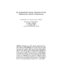## An Approximate Linear Approach for the Fundamental Matrix Computation2003-10-06
##### Authors
Guerra-Filho, Gutemberg
Aloimonos, Yiannis
##### Abstract
We introduce a new robust approach for the computation of the fundamental matrix taking into account the intrinsic errors (uncertainty) involved in the discretization process. The problem is modeled as an approximate equation system and reduced to a linear programming form. This approach is able to compute the solution set instead of trying to compute only a single vertex of the solution polyhedron as in previous approaches. Therefore, our algorithm is a robust generalization of the eight-point algorithm. The exact solution computation feasibility is proved for some pure translation motions, depending only on the distribution of the discretization errors. However, a single exact solution for the fundamental matrix is not feasible for pure rotation cases. The feasibility of an exact solution is decided according to an error distance between a nontrivial exact solution and the faces of the solution set.